# Inverse Of Diagonal Matrix

The diagonal matrix has diagonal elements only and if the diagonals are 1 then the matrix is called and identity matrix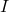. In this article, we discuss about diagonal matrix and properties. Then find the inverse of diagonal matrix.

### What is a diagonal matrix ?

A diagonal matrix is a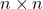matrix whose diagonal entries are non-zero and all other entries are zero. For example,

A = \begin{bmatrix}a_{11}&0&0\\0&a_{22}&0\\0&0&a_{33}\end{bmatrix}

The matrix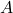above is a diagonal matrix whose diagonal entries are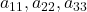and other entries are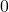.

### Some Interesting Properties of Diagonal Matrix

The diagonal matrix have some interesting properties explain in this section with examples.

Addition Or Multiplication of Diagonal Matrices

Addition or multiplication of two or more diagonal matrices of same order will give a diagonal matrix of same order.

Example #1

Letandbe two diagonal matrices of order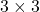.

A = \begin{bmatrix}2 & 0 & 0 \\0 & 7 & 0\\0 & 0 & 4\end{bmatrix} B = \begin{bmatrix}1 & 0 & 0 \\0 & 3 & 0\\0 & 0 & 5\end{bmatrix}

Therefore, addingandwill give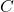A + B = C
C_{3\times3} = \begin{bmatrix}2 + 1 & 0 + 0 & 0 + 0\\0 + 0 & 7 + 3& 0+0\\ 0+0& 0 + 0&4+ 5\end{bmatrix} = \begin{bmatrix}3 & 0 & 0 \\0 & 10 & 0\\0 & 0 & 9\end{bmatrix}_{3\times3}

Example #2

Letand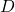be two diagonal matrices of order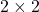.

C = \begin{bmatrix}4 & 0\\0 & 3\end{bmatrix}\hspace{5px} D =\begin{bmatrix} 1 & 0\\0 & 6\end{bmatrix}

Therefore, multiplying matrixandwill give

C \times D = F
\begin{aligned}
F_{2\times2} = \begin{bmatrix}4 + 0 & 0 + 0\\0 + 0&0 + 18\end{bmatrix}= \begin{bmatrix}4 & 0\\0 & 18\end{bmatrix}_{2\times2}
\end{aligned}

Multiplication Of Diagonal Matrix With Other Matrices

Letbe matrix of orderandbe a matrix of order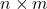.

Example #3

\begin{aligned}
&A = \begin{bmatrix}a_{11}&0&0\\0&a_{22}&0\\0&0&a_{33}\end{bmatrix}\\\\
&B = \begin{bmatrix}b_{11}&b_{12}\\b_{21}&b_{22}\\b_{31}&b_{32}\end{bmatrix}\\\\
&A \times B = \begin{bmatrix}a_{11}&0&0\\0&a_{22}&0\\0&0&a_{33}\end{bmatrix} \times \begin{bmatrix}b_{11}&b_{12}\\b_{21}&b_{22}\\b_{31}&b_{32}\end{bmatrix}\\\\
&A \times B = \begin{bmatrix}a_{11}b_{11}&a_{11}b_{12}\\a_{22}b_{21}&a_{22}b_{22}\\a_{33}b_{31}&a_{33}b_{32}\end{bmatrix}
\end{aligned}

Each element of diagonal matrixis multiplied with corresponding row elements of matrix. For example, element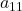from diagonal matrixis multiplied with all elements of first row in matrix.

Example #4

Another case of multiplication is when matrixof order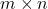is multiplied with a diagonal matrixof order.

Letbe a matrix of order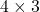.

C = \begin{bmatrix}c_{11}&c_{12}&c_{13}\\c_{21}&c_{22}&c_{23}\\c_{31}&c_{32}&c_{33}\\c_{41}&c_{42}&c_{43}\end{bmatrix}

andbe a diagonal matrix of order\begin{aligned}
&A = \begin{bmatrix}a_{11}&0&0\\0&a_{22}&0\\0&0&a_{33}\end{bmatrix}\\\\
&C \times A = \begin{bmatrix}c_{11}&c_{12}&c_{13}\\c_{21}&c_{22}&c_{23}\\c_{31}&c_{32}&c_{33}\\c_{41}&c_{42}&c_{43}\end{bmatrix}\times \begin{bmatrix}a_{11}&0&0\\0&a_{22}&0\\0&0&a_{33}\end{bmatrix}\\\\
&C \times A = \begin{bmatrix}c_{11}a_{11}+0+0&0+c_{12}a_{22}+0&0+0+c_{13}a_{33}\\c_{21}a_{11}+0+0&0+c_{22}a_{22}+0&0+0+c_{23}a_{33}\\c_{31}a_{11}+0+0&0+c_{32}a_{22}+0&0+0+c_{33}a_{33}\\c_{41}a_{11}+0+0&0+c_{42}a_{22}+0&0+0+c_{43}a_{33}\end{bmatrix}\\\\
&C \times A = \begin{bmatrix}c_{11}a_{11}&c_{12}a_{22}&c_{13}a_{33}\\c_{21}a_{11}&c_{22}a_{22}&c_{23}a_{33}\\c_{31}a_{11}&c_{32}a_{22}&c_{33}a_{33}\\c_{41}a_{11}&0+c_{42}a_{22}&c_{43}a_{33}\end{bmatrix}
\end{aligned}

When matrixis multiplied with diagonal matrixthen each element ofis multiplied with corresponding columns in matrix. For example, elementis multiplied with first column of the matrix.

Commutative Property of Multiplication in Between Diagonal Matrices

If two matricesandare diagonal matrices of same order, then the multiplication is commutative.

Example #5

Letbe a diagonal matrix of order.

A = \begin{bmatrix}3&0&0\\0&4&0\\0&0&5\end{bmatrix}

andbe a diagonal matrix of order.

\begin{aligned}
&A = \begin{bmatrix}-2&0&0\\0&1&0\\0&0&7\end{bmatrix}\\\\
&A \times B = \begin{bmatrix}3&0&0\\0&4&0\\0&0&5\end{bmatrix} \times \begin{bmatrix}-2&0&0\\0&1&0\\0&0&7\end{bmatrix}\\\\
&A \times B = \begin{bmatrix}-6&0&0\\0&4&0\\0&0&35\end{bmatrix}
\end{aligned}

Similarly,

\begin{aligned}
&B \times A = \begin{bmatrix}-2&0&0\\0&1&0\\0&0&7\end{bmatrix}\times \begin{bmatrix}3&0&0\\0&4&0\\0&0&5\end{bmatrix}\\\\
&A \times B = \begin{bmatrix}-6&0&0\\0&4&0\\0&0&35\end{bmatrix}
\end{aligned}

Therefore,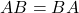for diagonal matrices is true.

Diagonal Matrix is Symmetric

Ifis a diagonal matrix of orderthen it is symmetric.

A = A^T

Example #6

Letbe a diagonal matrix of order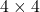.

A = \begin{bmatrix}a_{11}&0&0&0\\0&a_{22}&0&0\\0&0&a_{33}&0\\0&0&0&a_{44} \end{bmatrix}

When we take transpose of a matrix, then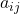becomes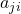, but for a diagonal matrix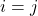, therefore,

\begin{aligned}
&A^T = \begin{bmatrix}a_{11}&0&0&0\\0&a_{22}&0&0\\0&0&a_{33}&0\\0&0&0&a_{44} \end{bmatrix} = A\\\\
&A = A^T
\end{aligned}

We conclude that the diagonal matrixis symmetric and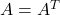is true.

Power of Diagonal Matrix

If a diagonal matrix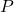is multiplied by itself k-times, then we can say that the matrixis raised to the power of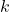.

Example #7

Letbe a diagonal matrix of order. Let matrixis raised to power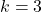then

\begin{aligned}
&P^3 = \begin{bmatrix}a & 0\\0 & b\end{bmatrix} \times \begin{bmatrix}a & 0\\0 & b\end{bmatrix}\times \begin{bmatrix}a & 0\\0 & d\end{bmatrix}\\\\
&P^3 = \begin{bmatrix}a^3 & 0\\0 & b^3\end{bmatrix}
\end{aligned}

Therefore,

P^k = \begin{bmatrix}a^k & 0\\0 & b^k\end{bmatrix}

### Invertible Diagonal Matrix

Any matrix is invertible if its determinant is not equal to 0 and it is a square matrix. The diagonal matrix is a square matrix, but it must have a non-zero entry in the main diagonal to be invertible.

If the main diagonal has a zero entry then it is a singular matrix for two reasons

1. It is not a square matrix
2. It has a zero determinant

Example #8

Let matrixbe a diagonal matrix of order.

A = \begin{bmatrix} a & 0 & 0\\0 & b & 0\\0 & 0 & 0\end{bmatrix}

Rule 1: Diagonal matrix must be a square matrix.

The last row of matrixis zero and must be deleted. The remaining two row is not a square matrix; therefore, not invertible.

Rule 2: The determinant of matrixshould be non-zero.

The minor matrix ofis

= \begin{bmatrix}0 & 0 &0\\0 & 0 & 0\\0 & 0 & ab\end{bmatrix}

The determinant of a diagonal matrixis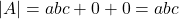if there are non-zero elements in the main diagonal.

Inverse of a Diagonal Matrix

The inverse of a diagonal matrix can be found by using the following equation.

A^{-1} = 1/|A|\times adj A

Example #9

Letbe a diagonal matrix of order.

A = \begin{bmatrix}a & 0 & 0\\0 & b & 0\\0 & 0 & c\end{bmatrix}

From the discussion above we know that the co-factor matrix of A is

= \begin{bmatrix}bc & 0 & 0\\0 & ac & 0\\0 & 0 & bc\end{bmatrix}

Since, matrixis symmetric, that is,.

Letbe a diagonal matrix of order.

A = \begin{bmatrix}a & 0 & 0\\0 & b & 0\\0 & 0 & c\end{bmatrix}

From the discussion above we know that the co-factor matrix of A is

= \begin{bmatrix}bc & 0 & 0\\0 & ac & 0\\0 & 0 & bc\end{bmatrix}

Since, matrixis symmetric, that is,.

adj A = \begin{bmatrix}A_{ij}\end{bmatrix}^T = \begin{bmatrix}bc & 0 & 0\\0 & ac & 0\\0 & 0 & bc\end{bmatrix}

Therefore,

\begin{aligned}
&A^{-1} = 1/abc\times \begin{bmatrix}bc & 0 & 0\\0 & ac & 0\\0 & 0 & bc\end{bmatrix}\\\\
&A^{-1} = \begin{bmatrix}1/a& 0 & 0\\0 &1/b& 0\\0 & 0 &1/c\end{bmatrix}
\end{aligned}

From the example it is clear that the inverse of a diagonal matrix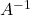contains reciprocal of each element of the diagonal matrix.

‘Example #10

Find the inverse of following diagonal matrix.

B = \begin{bmatrix}-3 & 0 & 0 \\0 & 2 & 0 \\ 0 & 0 & 5\end{bmatrix}

Solution :

Given the diagonal matrix.

B = \begin{bmatrix}-3 & 0 & 0 \\0 & 2 & 0 \\ 0 & 0 & 5\end{bmatrix}

We simply need to find the inverse of each diagonal element in the matrix.

Therefore,

B^{-1} = \begin{bmatrix}-1/3& 0 & 0 \\0 &1/2& 0 \\ 0 & 0 &1/5\end{bmatrix}

### Important Points To Remember

Here are some important points to remember.

• diagonal addition and multiplication with another diagonal matrix is commutative.
• diagonal matrix is symmetric.
• power of diagonal matrix is power of individual diagonal entries.
• invertible diagonal matrix has non-zero diagonal entries.
• inverse of a diagonal matrix is a matrix that has inverse of each corresponding element from the diagonal matrix.